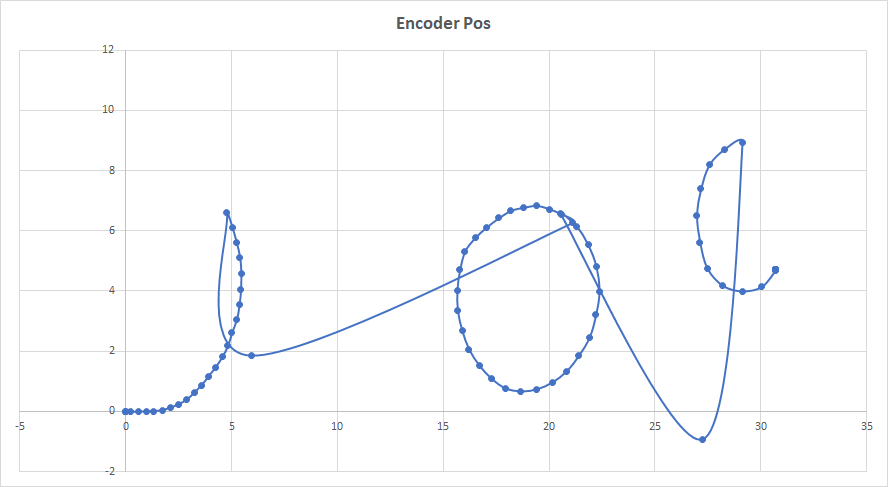# Encoder Odometry Problems

Hi, I have a problem with my robot and its encoders, basically, I'm trying to get the position in (X, Y) using encoders. I make a quadrature encoder and it generates 1440 tick per revolution and the wheel radius is 30 mm. Now, when I plot the calculated robot position through the encoder position, based on this code:

def get_position_using_encoders(self, dt, left_enc, right_enc, yaw=0, verbose=False):

# Calculate odometry

if self.enc_left != None:

dright_1 = (right_enc - self.enc_right)
dleft_1 = (left_enc - self.enc_left)

dright = dright_1 / self.tick_per_meter
dleft = dleft_1 / self.tick_per_meter

else:
dright = 0
dleft = 0

dxy_ave = (dright + dleft) / 2.0

self.dth += (dright - dleft) / self.wheel_track

y += dxy_ave * cos(self.dth + yaw)
x += dxy_ave * sin(self.dth + yaw)

self.enc_right = right_enc
self.enc_left = left_enc

return x, y


I have this graph:Honestly, I don't know why I have this.

Thanks

jarain78

edit retag close merge delete

Where physically does your encoder data come from? Is it simulated or really from encoders? Looks like corrupted data. Is it repeatable?

Hey, Billy, thanks for the comment. The encoder is real, I use the external interruption of an Arduino to count the encoder and send the count to my robot.

Regards

jarain78

Isolate your encoder data from the data transportation. Put a repeating number on the serial line between each encoder update and see if that number ever shows up corrupted or implement a simple CRC type check. Either your counting is failing or the communication is failing.

Hey, Billy, thanks for the comment. I solved my problem, it was like you said the data had been corrupted.

Thank you